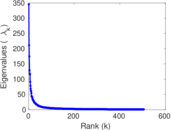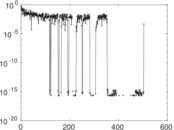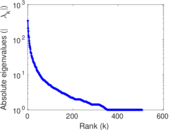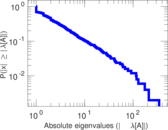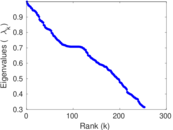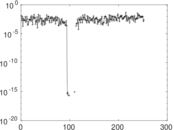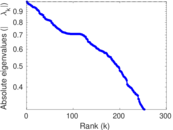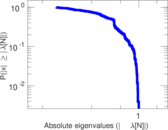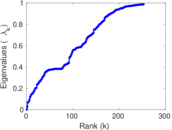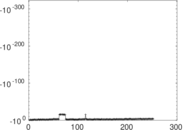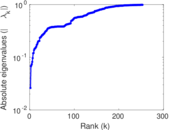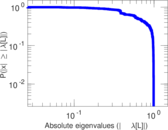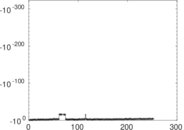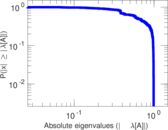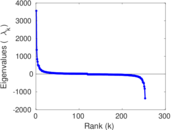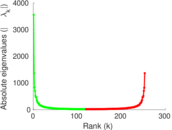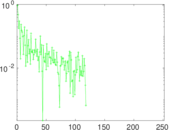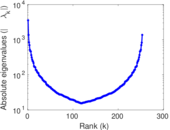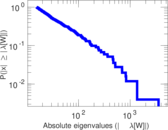# Wikipedia edits (om)

This is the bipartite edit network of the Oromo Wikipedia. It contains users and pages from the Oromo Wikipedia, connected by edit events. Each edge represents an edit. The dataset includes the timestamp of each edit.

 Code `om` Internal name `edit-omwiki` Name Wikipedia edits (om) Data source http://dumps.wikimedia.org/ AvailabilityDataset is available for download Consistency checkDataset passed all tests Category Authorship network Dataset timestamp 2017-10-20 Node meaning User, article Edge meaning Edit Network formatBipartite, undirected Edge typeUnweighted, multiple edges Temporal dataEdges are annotated with timestamps

## Statistics

 Size n = 3,582 Left size n1 = 630 Right size n2 = 2,952 Volume m = 19,420 Unique edge count m̿ = 7,835 Wedge count s = 777,752 Claw count z = 110,078,440 Cross count x = 15,956,457,500 Square count q = 928,008 4-Tour count T4 = 10,551,710 Maximum degree dmax = 2,122 Maximum left degree d1max = 2,122 Maximum right degree d2max = 489 Average degree d = 10.843 1 Average left degree d1 = 30.825 4 Average right degree d2 = 6.578 59 Fill p = 0.004 212 91 Average edge multiplicity m̃ = 2.478 62 Size of LCC N = 2,828 Diameter δ = 13 50-Percentile effective diameter δ0.5 = 3.647 59 90-Percentile effective diameter δ0.9 = 5.864 74 Median distance δM = 4 Mean distance δm = 4.335 98 Gini coefficient G = 0.835 647 Balanced inequality ratio P = 0.142 636 Left balanced inequality ratio P1 = 0.107 158 Right balanced inequality ratio P2 = 0.185 994 Relative edge distribution entropy Her = 0.806 564 Power law exponent γ = 2.913 57 Tail power law exponent γt = 2.061 00 Tail power law exponent with p γ3 = 2.061 00 p-value p = 0.000 00 Left tail power law exponent with p γ3,1 = 1.671 00 Left p-value p1 = 0.061 000 0 Right tail power law exponent with p γ3,2 = 2.221 00 Right p-value p2 = 0.000 00 Degree assortativity ρ = −0.338 432 Degree assortativity p-value pρ = 3.307 87 × 10−209 Spectral norm α = 345.166 Algebraic connectivity a = 0.026 094 6 Spectral separation |λ1[A] / λ2[A]| = 1.634 26 Controllability C = 2,203 Relative controllability Cr = 0.647 751

## Plots

### Fruchterman–Reingold graph drawing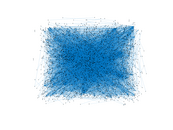### Degree distribution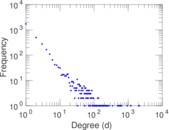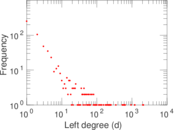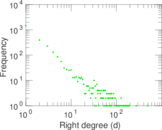### Cumulative degree distribution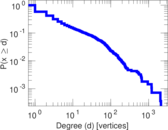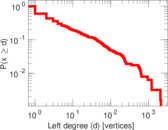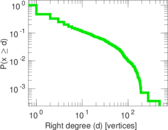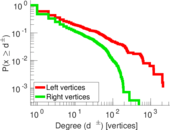### Lorenz curve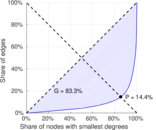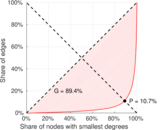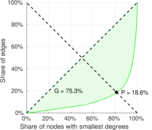### Spectral distribution of the adjacency matrix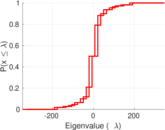### Spectral distribution of the normalized adjacency matrix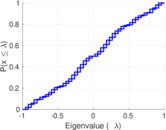### Spectral distribution of the Laplacian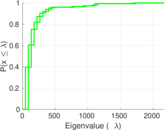### Spectral graph drawing based on the adjacency matrix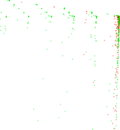### Spectral graph drawing based on the Laplacian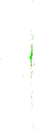### Spectral graph drawing based on the normalized adjacency matrix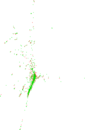### Degree assortativity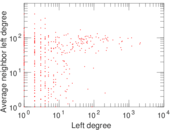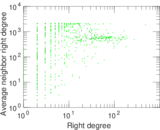### Zipf plot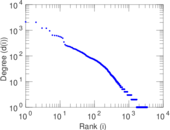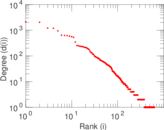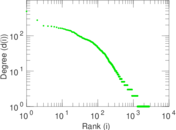### Hop distribution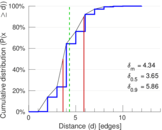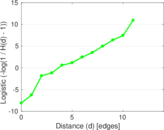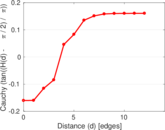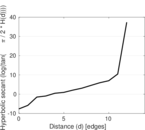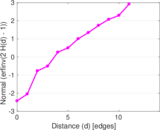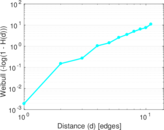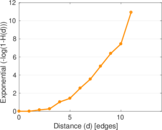### Double Laplacian graph drawing### Delaunay graph drawing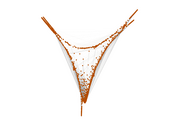### Edge weight/multiplicity distribution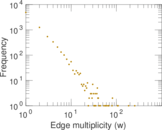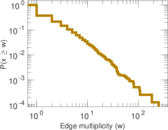### Temporal distribution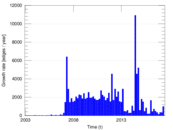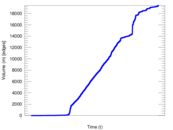### Temporal hop distribution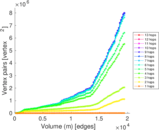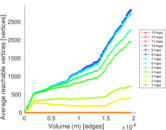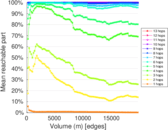### Diameter/density evolution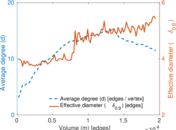### Matrix decompositions plots